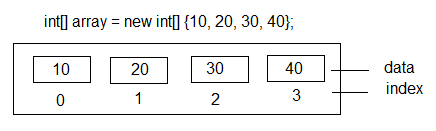VB.Net Tutorial

# How to VB.Net Arrays

An array is a data structure that allows you to store and manipulate a fixed-size collection of elements of the same type. Arrays provide a convenient way to work with multiple values of the same data type under a single variable name.## The commonly using functions are follows :

### Declaring an Array

To declare an array in VB.NET, you need to specify the data type of the elements and the size of the array. Here's an example of declaring an array of integers:

Dim numbers(4) As Integer

In this example, we declare an array called "numbers" that can hold 5 integer values (indexed from 0 to 4).

### Initializing an Array

After declaring an array, you can initialize it with values using various methods.

Inline initialization:
Dim numbers() As Integer = {1, 2, 3, 4, 5}
Initializing with a loop:
Dim numbers(4) As Integer For i As Integer = 0 To numbers.Length - 1 numbers(i) = i + 1 Next

### Accessing Array Elements

Array elements can be accessed using their index, which starts from 0 and goes up to the length of the array minus 1.

Dim numbers() As Integer = {1, 2, 3, 4, 5} Console.WriteLine(numbers(2)) ' Output: 3

### Modifying Array Elements

You can modify the values of array elements by assigning new values to them using their index.

Dim numbers() As Integer = {1, 2, 3, 4, 5} numbers(2) = 10 Console.WriteLine(numbers(2)) ' Output: 10

### Iterating through an Array

You can loop through the elements of an array using a For loop or a For Each loop. Here's an example using a For Each loop:

Dim numbers() As Integer = {1, 2, 3, 4, 5} For Each number As Integer In numbers Console.WriteLine(number) Next

### Array Properties and Methods

Arrays in VB.NET provide various properties and methods to work with. Here are a few commonly used ones:

1. Length Property: Returns the number of elements in the array.
2. Rank Property: Returns the number of dimensions in the array.
3. GetUpperBound Method: Returns the highest index value for a particular dimension.
Dim numbers() As Integer = {1, 2, 3, 4, 5} Console.WriteLine(numbers.Length) ' Output: 5 Console.WriteLine(numbers.Rank) ' Output: 1 Console.WriteLine(numbers.GetUpperBound(0)) ' Output: 4

### Multidimensional Arrays

VB.NET also supports multidimensional arrays, where you can have more than one dimension.

Dim matrix(2, 2) As Integer

In this example, we declare a 2-dimensional array called "matrix" with 3 rows and 3 columns.

## How to check if a value exists in an array ?

The provided VB.NET source code showcases a selection of frequently utilized functions.

Full Source VB.NET
Public Class Form1 Private Sub Button1_Click(ByVal sender As System.Object, ByVal e As System.EventArgs) Handles Button1.Click Dim numbers() As Integer = {1, 2, 3, 4, 5} ' Accessing Array Elements MessageBox.Show(numbers(2).ToString(), "Accessing Array Elements") ' Modifying Array Elements numbers(2) = 10 MessageBox.Show(numbers(2).ToString(), "Modifying Array Elements") ' Iterating through an Array Dim message As String = "" For Each number As Integer In numbers message += number.ToString() & vbCrLf Next MessageBox.Show(message, "Iterating through an Array") ' Array Properties and Methods MessageBox.Show(numbers.Length.ToString(), "Length Property") MessageBox.Show(numbers.Rank.ToString(), "Rank Property") MessageBox.Show(numbers.GetUpperBound(0).ToString(), "GetUpperBound Method") ' Multidimensional Arrays Dim matrix(2, 2) As Integer MessageBox.Show(matrix.GetLength(0).ToString() & " x " & matrix.GetLength(1).ToString(), "Multidimensional Arrays") End Sub End Class

### Conclusion

Arrays in VB.NET provide a powerful way to store and manipulate collections of values. They are widely used in various programming scenarios to store data efficiently and perform operations on multiple values simultaneously.